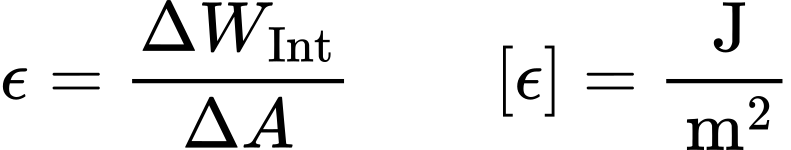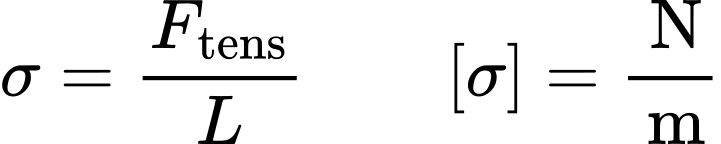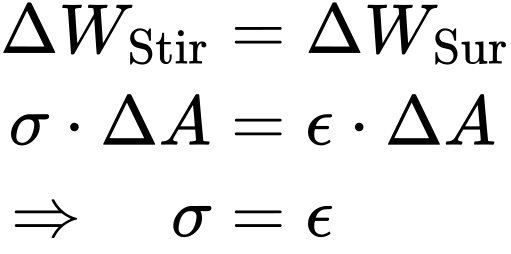Surface tension & surface energy# Surface tension & surface energy

A volume phase is a region without erratic changes in physical parameters. Contact between two different phases gives rise to a phase boundary. The contact area is called interface. There are five different types of interfaces: solid-solid, solid-liquid, solid-gaseous, liquid-liquid and liquid-gaseous (there is no gaseous-gaseous interface because all gases are miscible). The term surface is usually used for solid-gaseous and liquid-gaseous interfaces.

Note that even though the term interface suggests a sharp two dimensional boundary with an instantaneous change of the physical parameters, one finds in real systems, especially in the liquid-gaseous case, a three dimensional region in which the parameters change continuously (see figure 1). However the simplified assumption of a sharp interface is sufficient when coping with macroscopic interfacial phenomena.

Figure 1: Density profile at a liquid-gaseous interface

Contrary to the situation in ideal gases, the atoms/molecules of a solid or a liquid are held together by interaction forces. Inside of a phase the interaction forces on an atom/molecule equilibrate each other. At the interface forces from outside are missing, or significantly weaker, which results in a net force pointing into the phase (see figure 2). In order to bring an atom/molecule to the interface, work has to be done against this force. This work is stored in the atom/molecule as potential energy. Hence, an increase of the interface by ΔA requires an energy ΔWint. The interfacial energy is defined as the ratio:The surface energy of a liquid (liquid-gaseous interface) can be determined with a simple model experiment. Inside a U-shaped wire frame with a movable hoop at the open end a thin liquid film (e.g. soap solution) is spanned (see figure 3). The surface forces of the liquid film and the gravitational force of the movable hoop are in equilibrium. To displace the hoop by Δs and therefore increase the surface by ΔA=2bΔs (double the area of a rectangle because the film has a front and a back side) the force F has to be applied.

The liquid surface counteracts the surface increase through the force F by applying a tangential tension force Ftens on the hoop. This tension force is also called surface tension:For liquids the work ΔWhoop done on the hoop and the work ΔWint required for the surface increase are equal:Figure 3: model experiment on surface tension: Inside a U-shaped wire frame a liquid film is being stretched by the force F acting on a hoop

Thus, for liquids the surface tension and the surface energy are equal. The same considerations apply to liquid-liquid interfaces. However, note that the equality of interfacial energy and interfacial tension only applies to liquids because the atoms/molecules in liquid phases can be displaced without performing additional work. If the model experiment was carried out with a solid, the work to displace the atoms/molecules inside the solid phase would have to be taken into account. Hence, the simple equality of surface work and work done on the hoop would not hold true. In literature the tension and the energy are often denoted with the same symbol. Therefore only σ will be used for the interfacial tension/energy henceforth.

The surface and interfacial tension of liquids can be determined with an optical contact angle goniometer and drop shape analysis system of the OCA series using the pendant drop method. With a force tensiometer of the DCAT series the surface and interfacial tension of liquids can be determined via force measurements with a variety of measuring bodies like the Wilhelmy plate. Liquids with very low interfacial tension or very low density difference can be investigated with the spinning drop tensiometer of the SVT series. The surface energy of a solid can be determined by measurements of the contact angle and consideration of different models for the interaction between liquid and solid.

# Chapters

Using the following buttons you can navigate between the individual chapters of our knowledge-base Understanding Interfaces